We use cookies to improve our website and your experience when using it. Cookies used for the essential operation of the site have already been set. Continuing to use this site signifies your implied consent to the use of Cookies - pressing the “I Agree button” will remove this notice. To find out more about the cookies we use and how to delete them, see our "Privacy & Cookies" policy in the footer.

#1 SUPPLIER FOR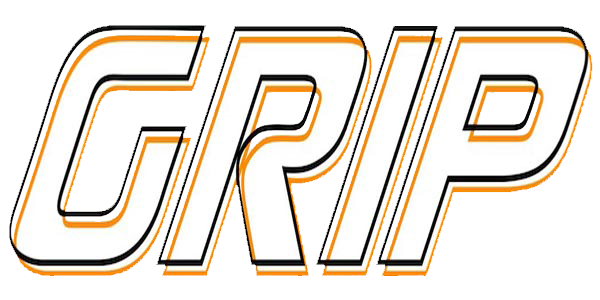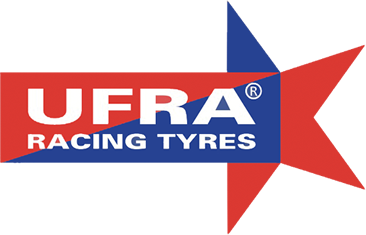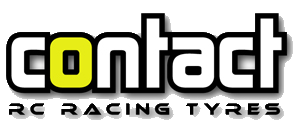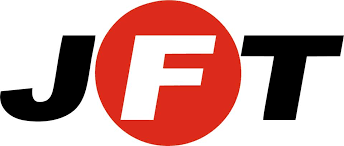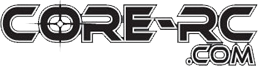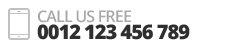×
x

Cart empty
Cart empty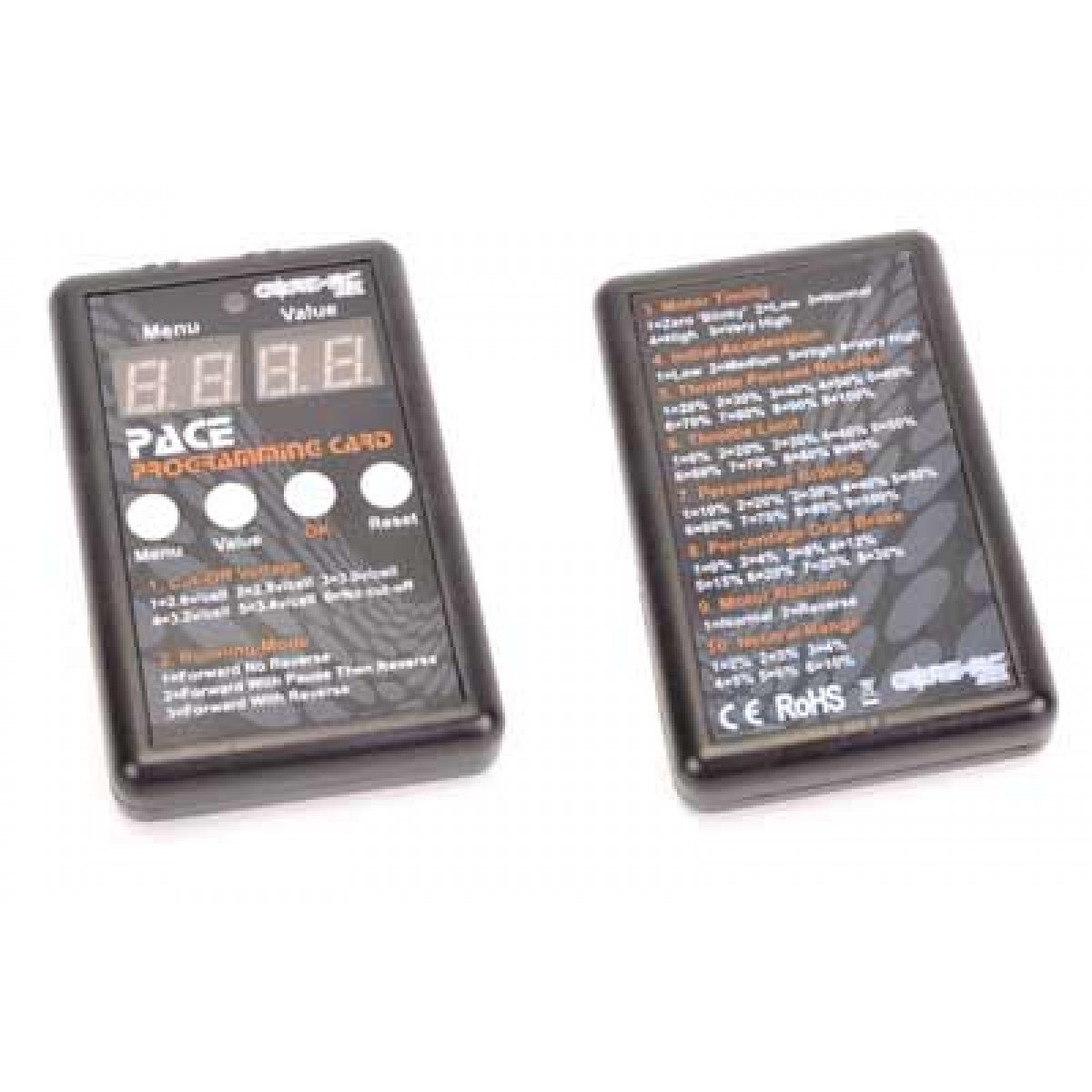# CR174 Stockcar & Saloon Legal

??? CR174 - PACE 45R Programming Card

PACE 45R Programming Card

CR174 - Optional program card available to allow multiple adjustments including;

1. Cut-Off Voltage
1=2.6v/cell 2=2.8v/cell 3=3.0v/cell 4=3.2v/cell 5=3.4v/cell 6=No cut-off
2. Running Mode
1=Forward No Reverse 2=Forward With Pause Then Reverse 3=Forward With Reverse
3. Motor Timing
1=Zero ???Blinky??? 2=Low 3=Normal 4=High 5=Very High
4. Initial Acceleration
1=Low 2=Medium 3=High 4=Very High
5. Throttle Percent Reverse
1=20% 2=30% 3=40% 4=50% 5=60% 6=70% 7=80% 8=90% 9=100%
6. Throttle Limit
1=0% 2=20% 3=30% 4=40% 5=50% 6=60% 7=70% 8=80% 9=90%
7. Percentage Braking
1=10% 2=20% 3=30% 4=40% 5=50% 6=60% 7=70% 8=80% 9=100%
8. Percentage Drag Brake
1=0% 2=4% 3=8% 4=12% 5=15% 6=20% 7=25% 8=30%
9. Motor Rotation
1=Normal 2=Reverse
10. Neutral Range
1=2% 2=3% 3=4% 4=5% 5=6% 6=10%
Array
Base price £9.50
Sales price £9.50
Total discount:
Description

??? CR174 - PACE 45R Programming Card PACE 45R Programming Card CR174 - Optional program card available to allow multiple adjustments including; 1. Cut-Off Voltage 1=2.6v/cell 2=2.8v/cell 3=3.0v/cell 4=3.2v/cell 5=3.4v/cell 6=No cut-off 2. Running Mode 1=Forward No Reverse 2=Forward With Pause Then Reverse 3=Forward With Reverse 3. Motor Timing 1=Zero ???Blinky??? 2=Low 3=Normal 4=High 5=Very High 4. Initial Acceleration 1=Low 2=Medium 3=High 4=Very High 5. Throttle Percent Reverse 1=20% 2=30% 3=40% 4=50% 5=60% 6=70% 7=80% 8=90% 9=100% 6. Throttle Limit 1=0% 2=20% 3=30% 4=40% 5=50% 6=60% 7=70% 8=80% 9=90% 7. Percentage Braking 1=10% 2=20% 3=30% 4=40% 5=50% 6=60% 7=70% 8=80% 9=100% 8. Percentage Drag Brake 1=0% 2=4% 3=8% 4=12% 5=15% 6=20% 7=25% 8=30% 9. Motor Rotation 1=Normal 2=Reverse 10. Neutral Range 1=2% 2=3% 3=4% 4=5% 5=6% 6=10%

#### Reviews

There are yet no reviews for this product.

### Welcome to Mardave

Welcome to Mardave RC Racing, the UK's number one 12th Oval RC car manufacturer!
Originally from Leicester, UK, Mardave produced the first ever RC modal car kit made in Europe back in the 60's. Now based in Norfolk, Mardave continue to produce models for all generations of RC racer buy ambien online.